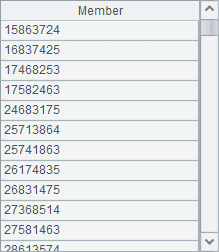# 当年销售额占到一半的前 n 个客户

l  问题l  思路

1.  为了以后能够方便的提取客户名字，先将合同表的客户字段替换为相应的客户记录。

2.  按要求，选出1998年的合同记录。

3.  对筛选出来的表按客户分组，这样每个客户的销售合同都被划归到一起了，同时再生成新的序表，求出每组的金额总和。

4.  将此序表按金额的降序排列。

5.  求出所有客户的总销售额，即年度总销售额值的一半。

6.  使用pselect函数，从上至下遍历每条记录，同时累计金额，直到金额达到总金额的一半时，停止遍历，就得到了大客户的数量n

7.  提取出前n个客户的名称，就是98年的大客户名单了。

l  代码

 A 1 =file("C:\\txt\\Contract.txt").import@t() 合同表 2 =file("C:\\txt\\Client.txt").import@t() 客户 3 >A2.keys(ID), A1.switch(Client,A2) 将合同表的客户字段替换成客户记录 4 =A1.select(year(SellDate)==1998) 筛选出98年的销售记录 5 =A4.group(Client;~.sum(Amount):Amount) 对表按客户分组，同时求出每组总金额 6 =A5.sort(-Amount) 按金额的降序排列 7 =A5.sum(Amount)/2 全年一半的销售额 8 =0 累计总金额的临时变量 9 =A6.pselect((A8=A8+Amount,A8>=A7)) 找到累计总金额达到一半的位置 10 =A6(to(A9)).(Client.Name) 提取出名字，就是该年的大客户了

l  结果